# Publicon in Action

## Extracts from Students’ Work

Slovak University of Technology, Faculty of Mechanical Engineering

Abstract

This chapter presents examples of work by students which illustrates the appearance of basic elements in a Publicon document. Authors of the extracts are the students of Slovak  University of Technology, Faculty of Mechanical Engineering.

### 1.  Numerical Methods and Mechanical Engineering

CALCULATING THE INTERNAL FORCES OF A TRUSS BRIDGE CONSTRUCTION

#### 1.1.  Introduction to the technical background

In architecture and structural engineering, a truss is a structure comprising one or more triangular units constructed with straight, slender members whose ends are connected at joints.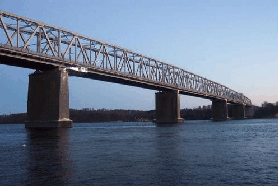Figure 1.

A plane truss is one where all the members and joints lie within a 2-dimensional plane, while a space truss has members and joints extending into 3 dimensions. A necessary (but not sufficiant) condition for stability is: $m\ge \text{}2j–r$ ($m$ - number of trusses, $j$ - number of joints, $r$- number of reactions). When $m=2j\text{−}3$, the truss is said to be statically determinate.   In order for a truss with pin-connected members to be stable, it must be entirely composed of triangles.
When  the external loads and the geometry of a truss are known, we can create the   equilibrium equations. This method of solution is called the joints method and more in detail can be seen in the solution of the example... 

###### Assumption for members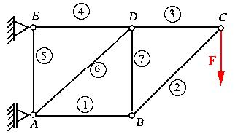Figure 2.

Each member represents an internal bond between two point masses.
The bond removes one degree of freedom from the mass points, in the direction of the member axis.
Then, the bonds are substituted by  internal bond reactions, which are called the axial forces in members. They are noted as ${P}_{i}$, where $i$ is the number of the member.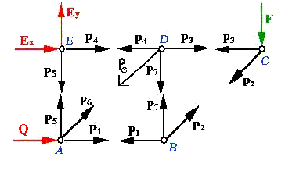Figure 3.

###### Notation and sign convention for trusses

The amplitude and orientation of internal forces in a truss depends on the geometry of the structure, loaded by external forces and the positioning of supports. Positive (tensile) axial force is oriented out of an imaginary cut in a member or out of a mass point. A negative (compressive) axial force is oriented into an imaginary cut or mass point. 

To calculate the forces in a structure we have to derive equilibrium equations for every joint. 

Corresponding equations :

${F}=20\mathrm{kN}$

$A:$

 ${F}_{x}:{Q}+{P}_{1}+{P}_{6}\mathrm{cos\alpha }=0$ (1)
 ${F}_{y}:\text{}{P}_{5}+{P}_{6}\mathrm{sin\alpha }=0$ (2)

$B:$

 ${F}_{x}:-{P}_{1}+{P}_{2}\mathrm{cos\alpha }=0$ (3)
 ${F}_{y}:\text{}{P}_{7}+{P}_{2}\mathrm{sin\alpha }=0$ (4)

$C:$

 ${F}_{x}:-{P}_{3}-{P}_{2}\mathrm{cos\alpha }=0$ (5)
 ${F}_{y}:\text{}-{P}_{2}\mathrm{sin\alpha }={F}$ (6)

$D:$

 ${F}_{x}:{P}_{3}-{P}_{4}-{P}_{6}\mathrm{cos\alpha }=0$ (7)
 ${F}_{y}:\text{}-{P}_{7}-{P}_{6}\mathrm{sin\alpha }=0$ (8)

$E:$

 ${F}_{x}:{P}_{4}+{E}_{x}=0$ (9)
 ${F}_{y}:{E}_{y}-{P}_{5}=0$ (10)

A system of 10 linear equations in 10 variables has to be solved. Assuming that angle is $45\text{˚}$, we obtain the coefficients for the tables and $A$ matrix in the formula $A.x=b$, where $x=\left\{{P}_{1},{P}_{2},{P}_{3},{P}_{4},{P}_{5},{P}_{6},{P}_{7},{E}_{x},{E}_{y},A\right\}$ .

Table 1. Equations left sides coefficients

 Number ${P}_{1}$ ${P}_{2}$ ${P}_{3}$ ${P}_{4}$ ${P}_{5}$ ${P}_{6}$ ${P}_{7}$ ${E}_{x}$ ${E}_{y}$ $Q$ 1 1 0 0 0 0 0,707 0 0 0 1 2 0 0 0 0 1 0,707 0 0 0 0 3 -1 0,707 0 0 0 0 0 0 0 0 4 0 0,707 0 0 0 0 1 0 0 0 5 0 -0,707 -1 0 0 0 0 0 0 0 6 0 -0,707 0 0 0 0 0 0 0 0 7 0 0 1 -1 0 -0,707 0 0 0 0 8 0 0 0 0 0 -0,707 -1 0 0 0 9 0 0 0 1 0 0 0 1 0 0 10 0 0 0 0 -1 0 0 0 1 0

Table for the matrix $A$

Table 2. Equations right sides

 Number F 1 0 2 0 3 0 4 0 5 0 6 20 000 7 0 8 0 9 0 10 0

Table for the vector $b$

By this means matrices will look like this.

Matrix $A$:

$A=\left(\begin{array}{cccccccccc}1& 0& 0& 0& 0& 0.707& 0& 0& 0& 1\\ 0& 0& 0& 0& 1& 0.707& 0& 0& 0& 0\\ -1& 0.707& 0& 0& 0& 0& 0& 0& 0& 0\\ 0& 0.707& 0& 0& 0& 0& 1& 0& 0& 0\\ 0& -0.707& -1& 0& 0& 0& 0& 0& 0& 0\\ 0& -0.707& 0& 0& 0& 0& 0& 0& 0& 0\\ 0& 0& 1& -1& 0& -0.707& 0& 0& 0& 0\\ 0& 0& 0& 0& 0& -0.707& -1& 0& 0& 0\\ 0& 0& 0& 1& 0& 0& 0& 1& 0& 0\\ 0& 0& 0& 0& -1& 0& 0& 0& 1& 0\end{array}\right)$

Vector $b$:

$b=\left(\begin{array}{c}0\\ 0\\ 0\\ 0\\ 0\\ 20000\\ 0\\ 0\\ 0\\ 0\end{array}\right)$

#### 1.2.   Solution via Numerical Methods

For solving the System of linear equations we can use Direct (LU-decomposition, Choleski, QR-decomposition) or Iteration (Jacobi, Gauss-Seidel, SOR method) methods.

##### 1.2.1.  Direct Methods

From direct methods we choose LU-decomposition ... 

Entries of the matrix L and the matrix U are computed under the formulas1:

${U}_{i,j}={A}_{i,j}-\sum _{r=1}^{i-1}{L}_{i,r}{U}_{r,j}$

${L}_{i,j}=\frac{1}{{U}_{j,j}}\left({A}_{i,j}-\sum _{s=1}^{j-1}\sum _{i=j+1}^{n}{L}_{i,s}{U}_{s,j}\right)......$

##### 1.2.2.  Iteration Methods

If we want to use the iteration methods to solve the problem we have to keep on these rules:

• The matrix $A$ must be a square matrix
• The matrix $A$ must be strictly diagonally dominant
• The matrix must be non-singular (i.e. have a non-zero determinant)

The matrix $A$ isn’t strictly diagonally dominant so we have to apply linear modification to achieve this. The form of strictly diagonally dominant matrix $A$ will be... 

### 2.  Statistics

ANALYZING THE WEIGHT DISTRIBUTION OF PORTORICO CIGARS

#### 2.1.   Introduction

....  In this section we use data that has been collected about the weight of cigars produced by Portorico. A sample of 300 cigars has been used in the analysis. Our main task is to organize and analyze the data using the method of Shewhart’s control chart. This chart contains the central line (CL - main line), the upper control limit line (UCL), and the lower control limit line (LCL). These parameters are calculated using the formulas:

$\mathrm{CL}=\text{}\stackrel{_}{\stackrel{_}{X}}=\frac{\sum _{i=1}^{k}{\stackrel{_}{X}}_{n}}{k}$

$\mathrm{UCL}=\stackrel{_}{\stackrel{_}{X}}+{A}_{2}\stackrel{_}{R}$

$\mathrm{LCL}=\stackrel{_}{\stackrel{_}{X}}-{A}_{2}\stackrel{_}{R}$

The histogram of all measured data can be seen in the Figure 4.2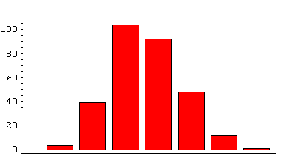Figure 4. ${3}^{}$

###### First analysis:  Nonexcluded data

In the first case we divided data into 30 subgroups of subgroup size 5 and basic parameters were computed .

X-bar and Range - Initial Study for weight with the subgroup size 5

... Outliers are colored by red stars in the graph. As one can see,  there is 1 outlier in the X-bar chart (Figure 5)2 and none in the Range chart (Figure 6)2 .  The outlier is excluded in the next process and ...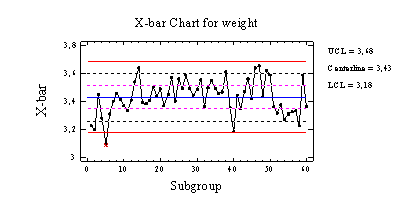Figure 5.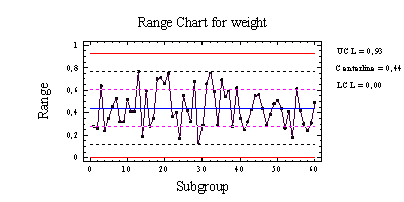Figure 6.

TRAJECTORY OF THE CENTRE OF GRAVITY

Figure 7 2shows the trajectory of the centre of gravity measured on ...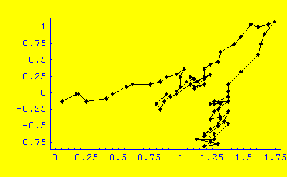Figure 7. ${7}^{}$

### 3.  Summary

Publicon belongs to the new editors focussed mainly on web publishing. Its main advantage consists in user friendly button click interface and MathML coding used for mathematical and chemical notation.  Although some aspects of its design are less than perfect, Publicon is easy to be use allowing the importing and exporting of objects  from and to other software products withot the loss of information.

This document has been written under the Article2  style sheet,  and demonstrates

• various kinds of cells
• document structuring - title, subtitle, author, abstract, section, subsection, subsubsection, endnote, reference
• cell forming - text, equation, numbered equation, graphics (figure, captions), table, table note
• imported graphics
• created tables, data plots, cross references, reference database entries, notes, references
• automated numbering of sections, tables, figures, pictures, notes, and references

### Notes

 1 Originally, the formulas were edited as display formulas in Mathematica notebook and then they were copied into the Publicon.

 2 Cross Reference

 3 The histogram was created as a bar chart in Publicon using prepared CSV data file.

 2 Cross Reference

 2 Cross Reference

 2 Cross Reference

 7 The graph of the trajectory was created in Publicon as a Line chart from prepared CSV data file. The  background was set to the yellow colour.

### References

  Šuhajdová, J. (2008). Solving The Internal Forces Of The Truss Bridge Construction (Student's Technical Work, Slovak University of Technology in Bratislava, Faculty of Mechanical Engineering, Bratislava).

  Marčák, M. (2008). Cane As System Of Mass Points (Student's Technical Work, Slovak University of Technology in Bratislava, Faculty of Mechanical Engineering, Bratislava).

  Čelko, M. (2008). Truss System (Student's Technical Work, Slovak University of Technology in Bratislava, Faculty of Mechanical Engineering, Bratislava).

  Darula, M. (2008). Methods of Solving Systems of Linear Equations Using Mathemathica Software (Student's Technical Work, Slovak University of Technology in Bratislava, Faculty of Mechanical Engineering, Bratislava).

  Baranek, J. (2003). Analyzing Of Weight Of Cigars (Student's Technical Work, Slovak University of Technology in Bratislava, Faculty of Mechanical Engineering, Bratislava).